# Length Node

Access: NodesMathLength

The Length node takes an input vector and outputs the length (or magnitude) of the vector. The following formula is used to calculate the length of the vector: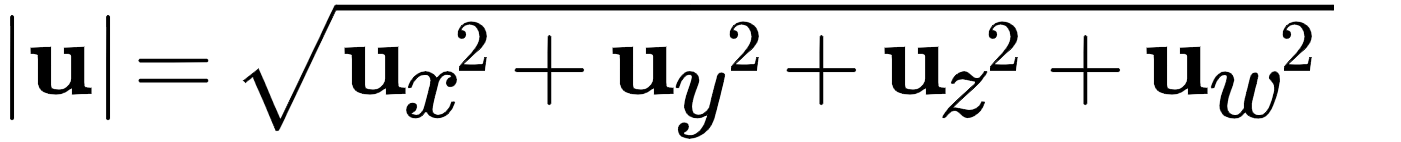Where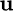is the vector [xyzw] and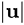is the length of the vector.

The output is a vector and the alpha channel always has a value of 1.0. Therefore the output is in the format:

[1 ]

Tip:  For more information on the vector length equation solution, see the Vector Magnitude Calculator by OnlineMSchool.

# Length Node Examples

 Input Calculation Output= [RGBA] = [3 1 2 0]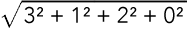= 3.742 [3.742 3.742 3.742 1.0]= [RGBA] = [10 5 4 0.5]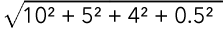= 11.885 [11.885 11.885 11.885 1.0]= [RGBA] = [12 25 6 1]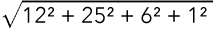= 28.390 [28.390 28.390 28.390 1.0]

# Length Node Inputs

 Input The vector to calculate the length of.# Length Node Outputs

 Outputs The length of the vector.# Length Node Properties

 R checkbox When checked, the red component is included in the length calculation. G checkbox When checked, the green component is included in the length calculation. B checkbox When checked, the blue component is included in the length calculation. A checkbox When checked, the alpha component is included in the length calculation. Note:  When the alpha is checked, its value is included in the length calculation however the alpha output will always be 1.0.